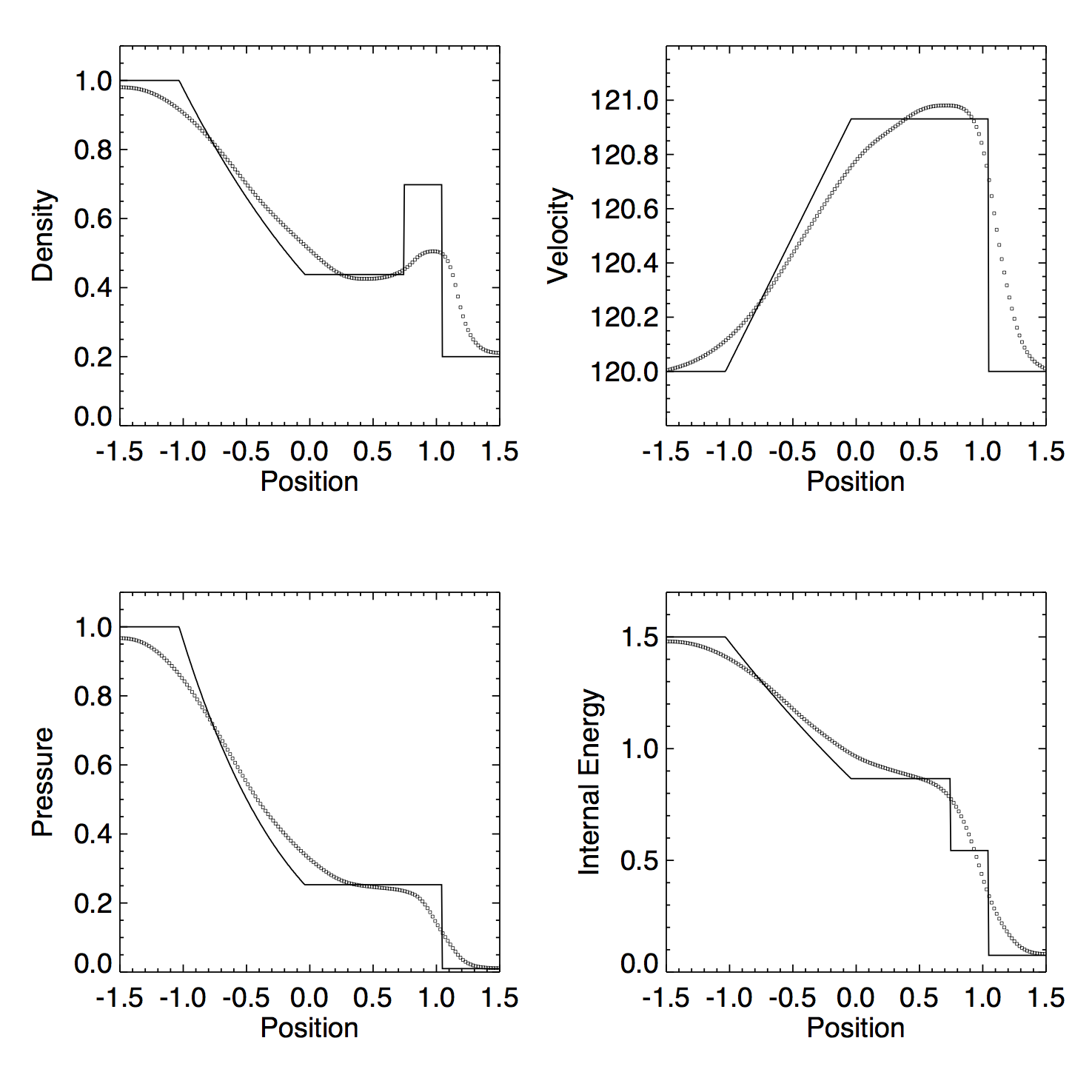# PLM tests

## October 5, 2015

What happens if we look at the same two high mach number tests from the previous posts (the square wave test and the shock tube test), but use a piecewise linear reconstruction?

Figure 1a: PLMC, exact solver, no dual energy, $v \approx 800 c_s$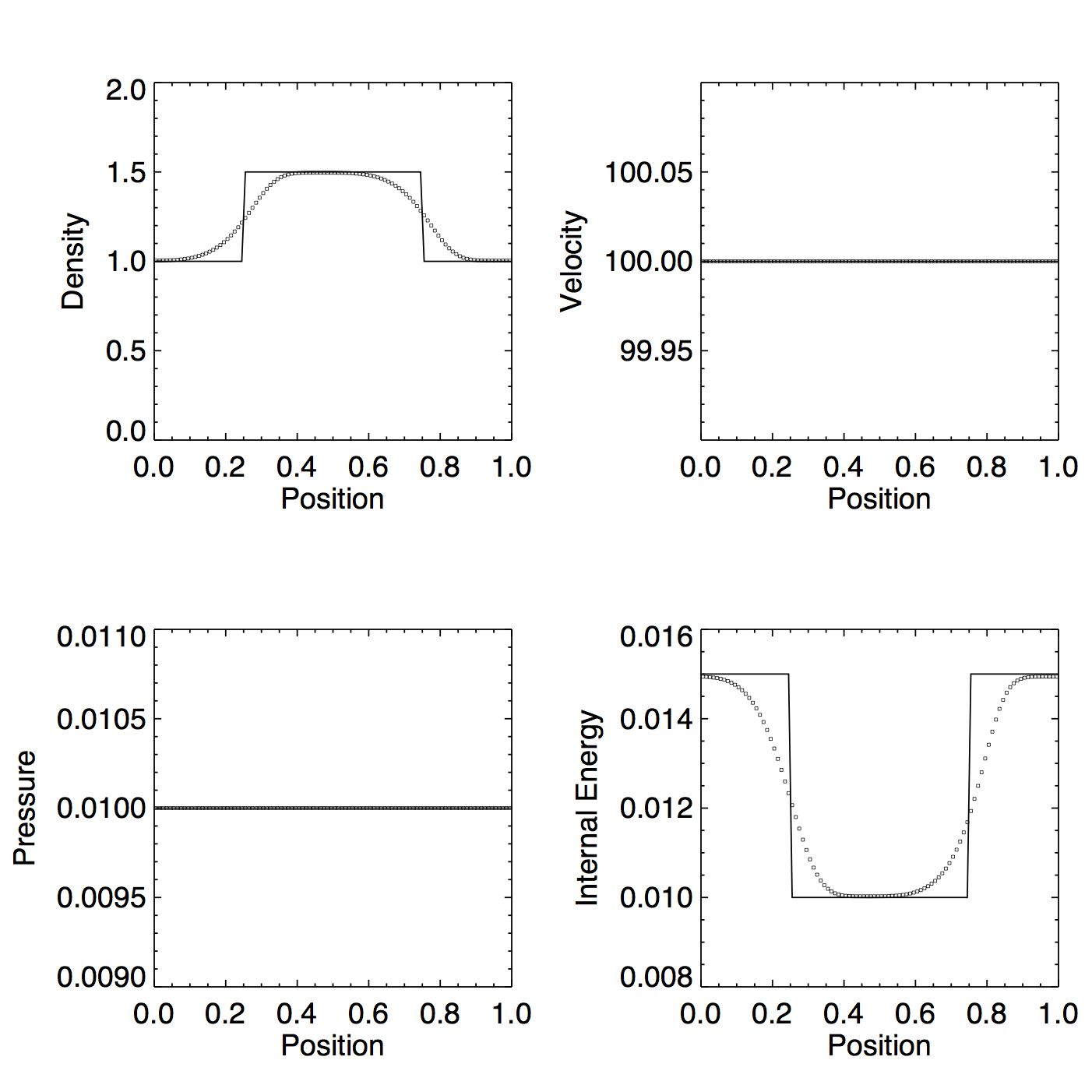Figure 1b: Same as Figure 1a but with dual energy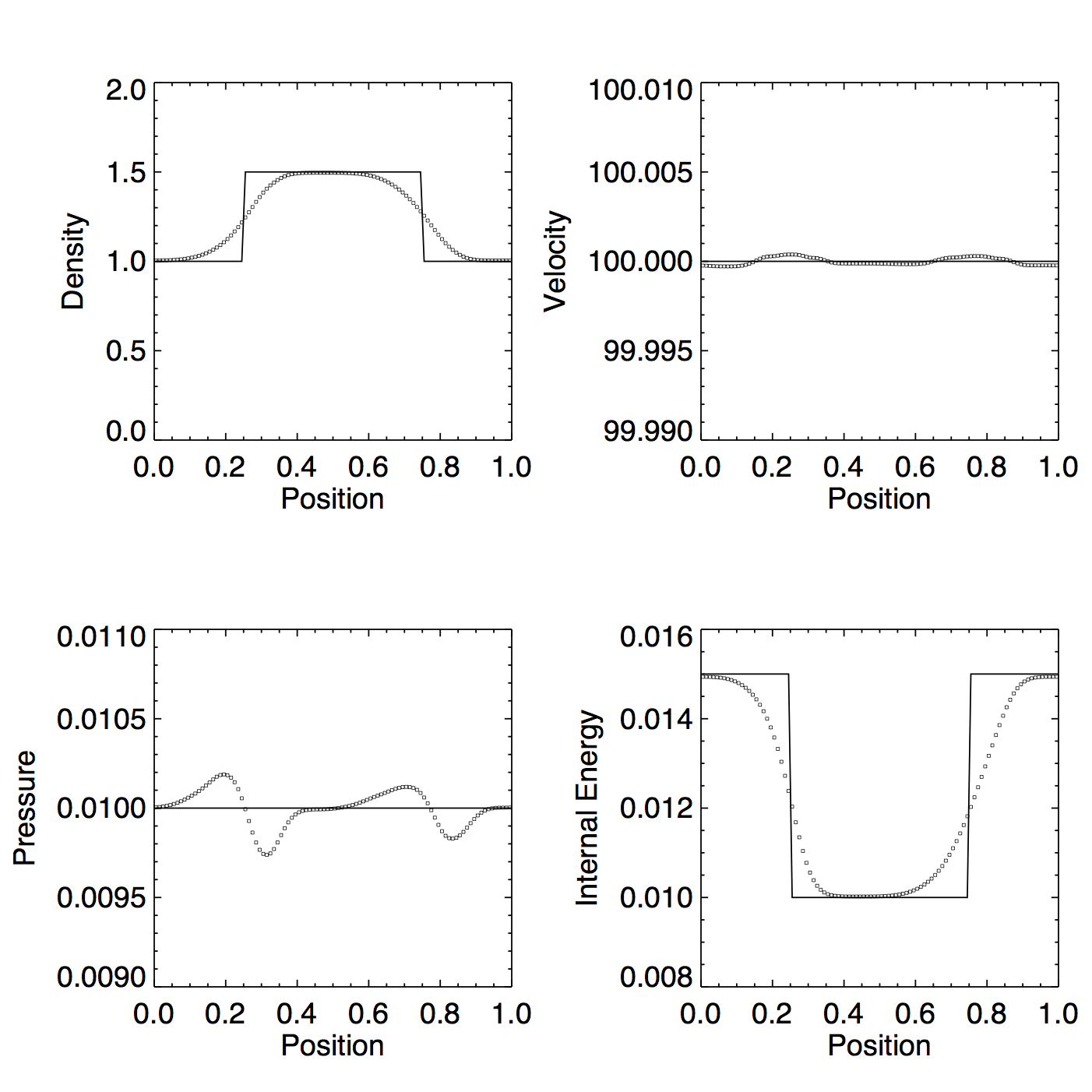Figure 2a: PLMP, exact solver, no dual energy, $v \approx 800 c_s$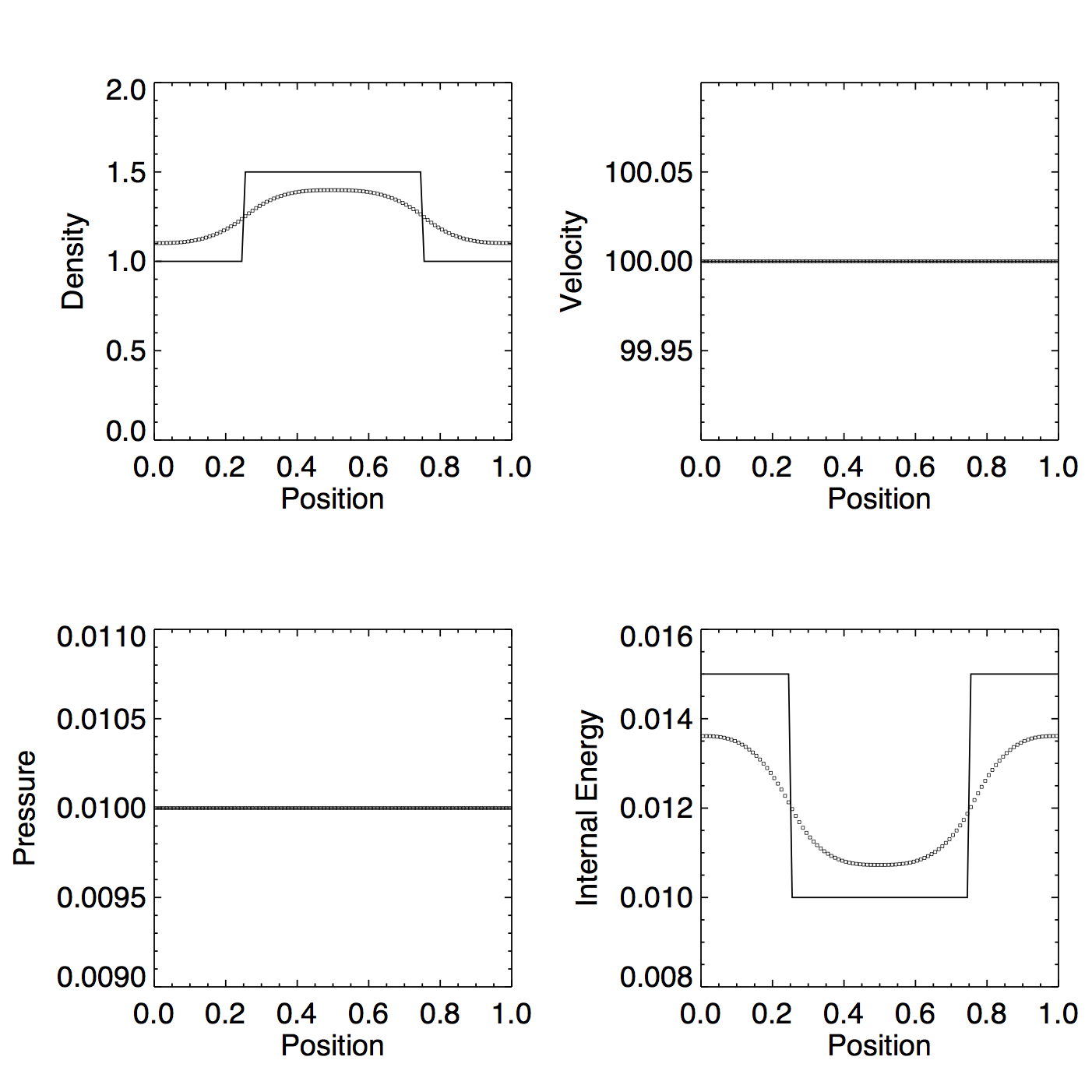Figure 2b: Same as Figure 2a but with dual energy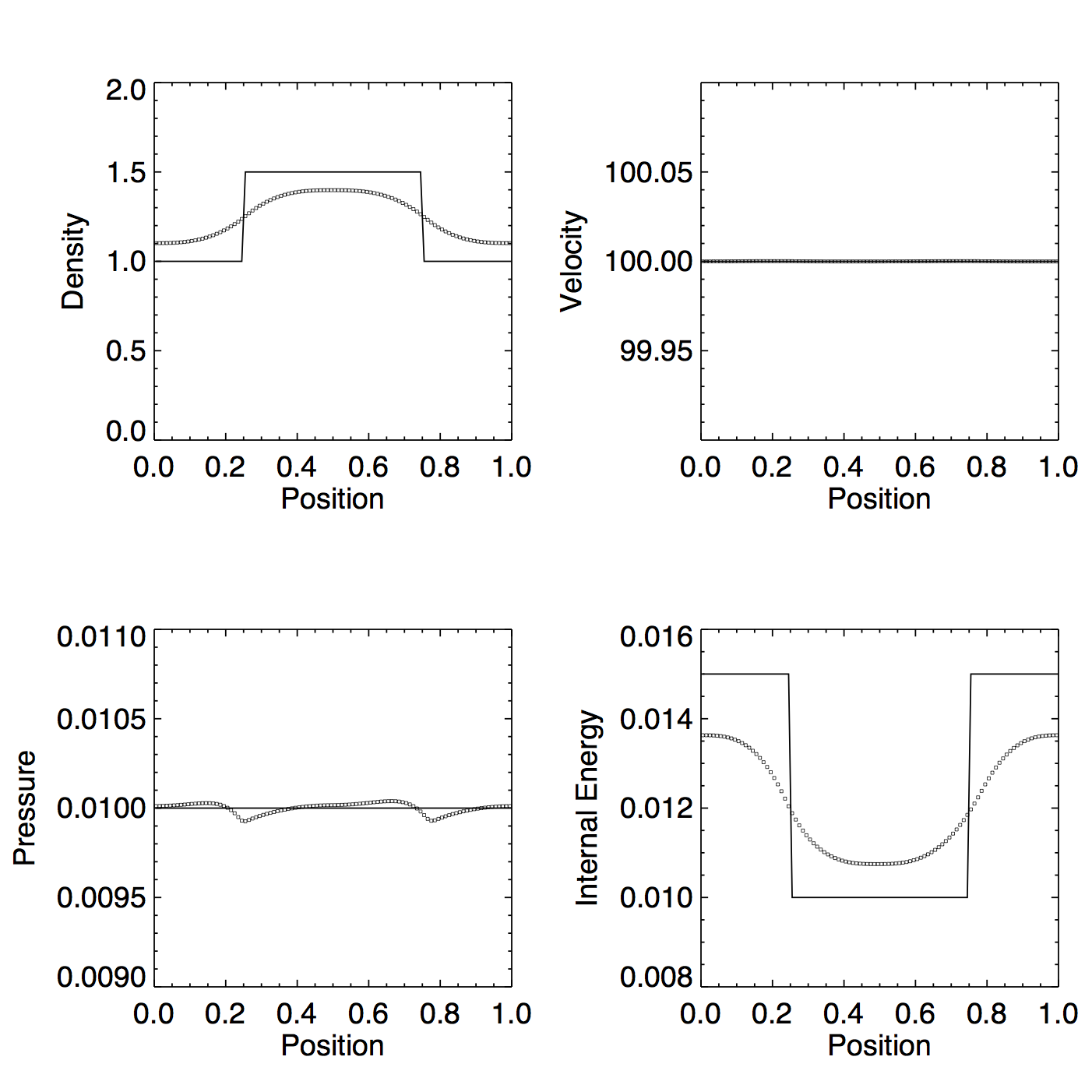Figure 3a: PLMC, exact solver, no dual energy, $v \approx 100 c_s$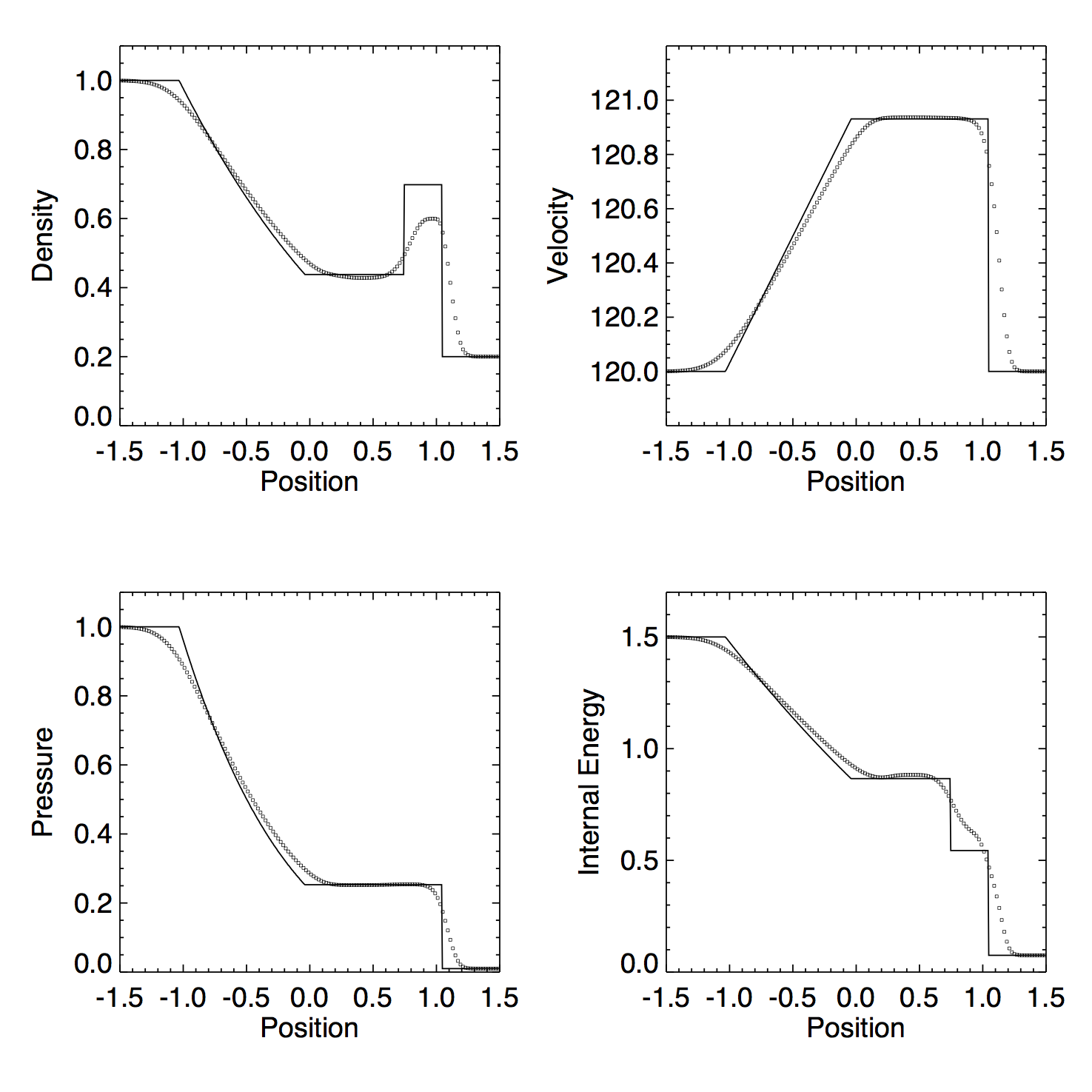Figure 3b: Same as Figure 3a but with dual energy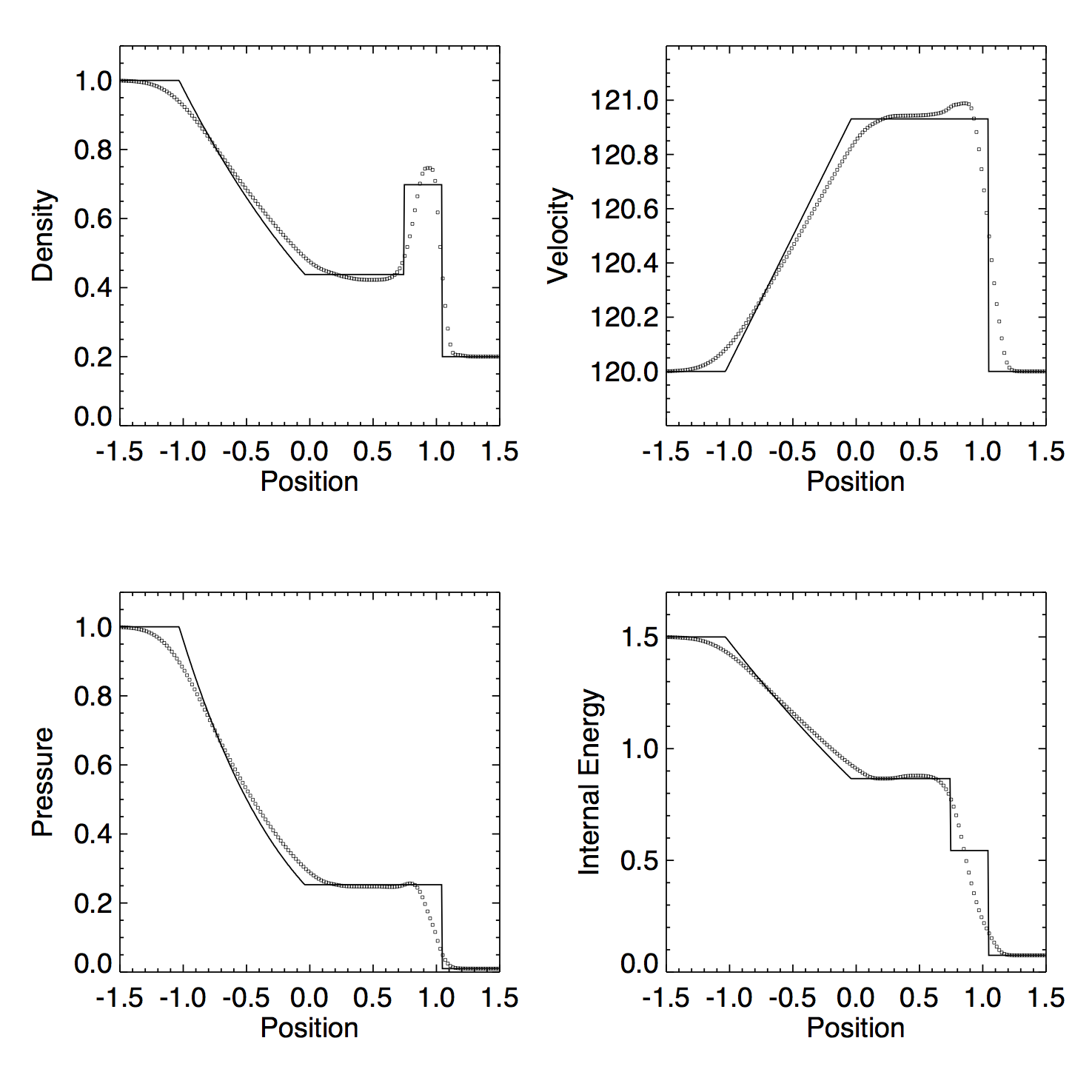Figure 4a: PLMP, exact solver, no dual energy, $v \approx 100 c_s$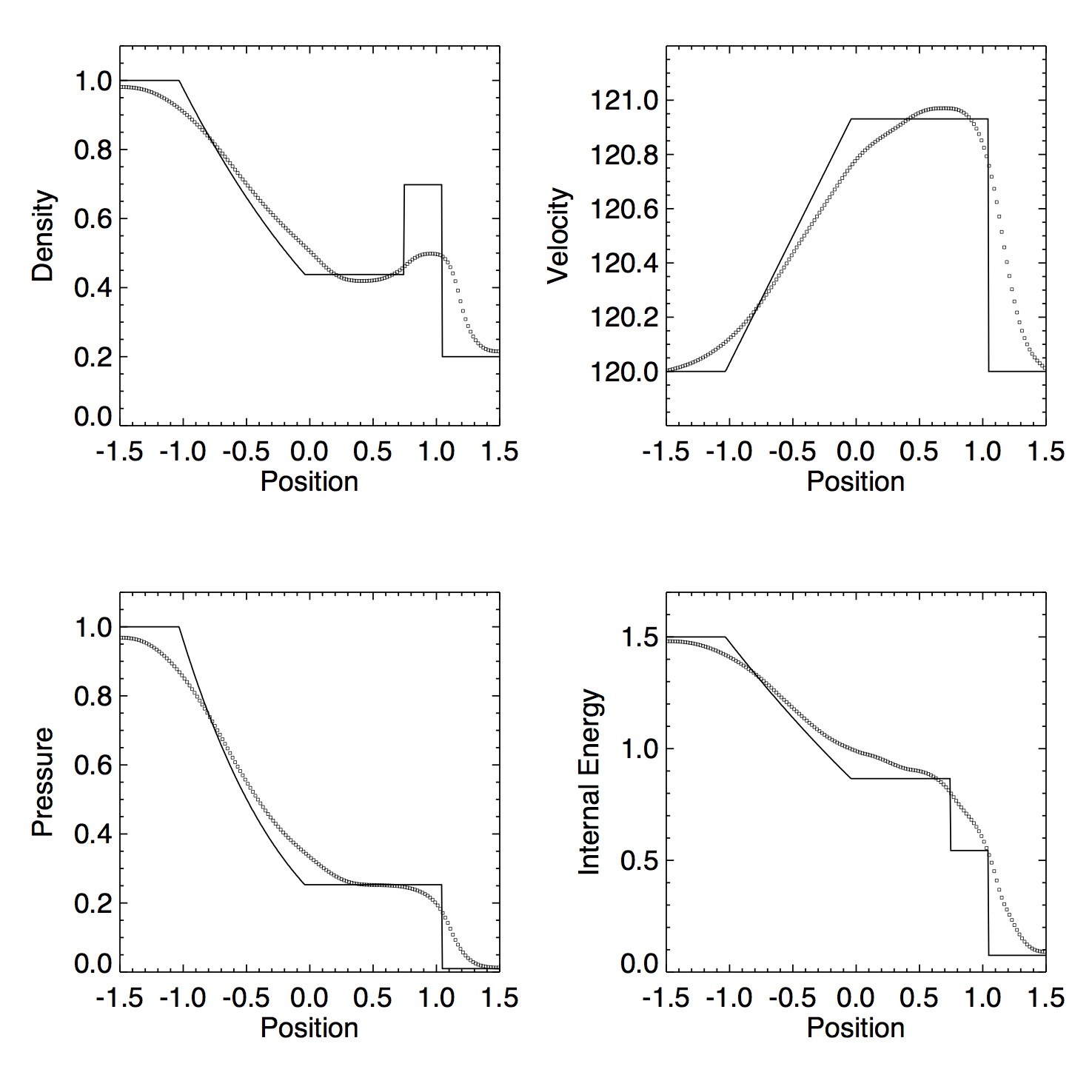Figure 4b: Same as Figure 4a but with dual energy